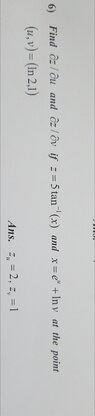# Calc Solved! Get Quick Help With Your Calculus Q

• MHB
• AHMED2021

#### AHMED2021

please I need solve of this question very quickly

#### Attachments

•203.7 KB · Views: 37
Then you had better get started! Can we at least assume you are taking a Calculus class?

Do you know the "chain rule"?

f is a function of x and x itself is a function of u and v so
$\frac{\partial f}{\partial u}= \frac{df}{dx}\frac{\partial x}{\partial u}$ and $\frac{\partial f}{\partial v}= \frac{df}{dx}\frac{\partial x}{\partial v}$.

f(x)= arctan(x) so $\frac{df}{dx}= \frac{1}{x^2+ 1}$

$x= e^u+ ln(v)$ so $\frac{\partial x}{\partial u}= e^u$ and $\frac{\partial x}{\partial v}= \frac{1}{v}$.

Last edited: